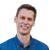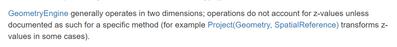# Bug with GeometryEngine.Intersection in ESRI.ArcGIS runtime version 100.14.1

309
2
12-02-2022 03:01 AMby
New Contributor II

Hello everyone,

I discovered a bug in the ESRI.ArcGIS runtime version 100.14.1.

More specifically, it's about the GeometryEngine.Intersection function call, which constructs an intersection between two geometries.

This bug does not appear in ESRI.ArcGIS runtime version 100.9.0.

I wrote a small sample program that shows this bug. I pasted it below.

``````using Esri.ArcGISRuntime.Geometry;

namespace EsriIntersection
{
public static class MySpatialReferences
{
public static SpatialReference Mercator = new SpatialReference(102100);
public static SpatialReference Wgs84 = new SpatialReference(4326);
}

public class GeoPoint
{
public double Latitude;
public double Longitude;
public double Altitude;

public GeoPoint(double latitude, double longitude, double altitude)
{
Latitude = latitude;
Longitude = longitude;
Altitude = altitude;
}
}

public class Program
{
private static void Main()
{
List<GeoPoint> legGeoPoints = CreateLegGeoPoints();
Geometry legGeometry = CreateLine(legGeoPoints);

List<GeoPoint> baseGeometryGeoPoints = CreateBaseGeometryGeoPoints();
Geometry baseGeometry = CreatePolygon(baseGeometryGeoPoints);

Geometry resultPolygon = GeometryEngine.Intersection(baseGeometry, legGeometry);

{
Console.WriteLine("Altitude: {0}", part.StartPoint.Z);
}
}

private static List<GeoPoint> CreateLegGeoPoints()
{
List<GeoPoint> legGeoPoints = new List<GeoPoint>();
GeoPoint p1 = new GeoPoint(48.4165771802278, 11.4771135568153, 564.558573541593);
GeoPoint p2 = new GeoPoint(48.4079168013471, 11.4661978649996, 564.558573541593);
return legGeoPoints;
}

private static List<GeoPoint> CreateBaseGeometryGeoPoints()
{
List<GeoPoint> baseGeometryGeoPoints = new List<GeoPoint>();
GeoPoint p1 = new GeoPoint(48.4258287904376, 11.4613346053484, 0);
GeoPoint p2 = new GeoPoint(48.4258827480755, 11.4615642474136, 0);
GeoPoint p3 = new GeoPoint(48.420352090187, 11.4784902184524, 0);
GeoPoint p4 = new GeoPoint(48.4202261890318, 11.4785847769498, 0);
GeoPoint p5 = new GeoPoint(48.40589144322, 11.4728032002502, 0);
GeoPoint p6 = new GeoPoint(48.4058105067631, 11.4725465414715, 0);
GeoPoint p7 = new GeoPoint(48.4125372256258, 11.4522704979526, 0);
GeoPoint p8 = new GeoPoint(48.4126901056, 11.4521894478119, 0);

return baseGeometryGeoPoints;
}

private static Geometry CreatePolygon(List<GeoPoint> geoPointList)
{
IEnumerable<MapPoint> mapPoints = geoPointList
.Select(CreateMapPointFromGeoPoint);

return new Polygon(mapPoints);
}

private static Geometry CreateLine(List<GeoPoint> geoPointList)
{
IEnumerable<MapPoint> mapPoints = geoPointList
.Select(CreateMapPointFromGeoPoint);

return new Polyline(mapPoints);
}

private static MapPoint CreateMapPointFromGeoPoint(GeoPoint geoPoint)
{
MapPoint mapPoint = new MapPoint(geoPoint.Longitude, geoPoint.Latitude, geoPoint.Altitude, MySpatialReferences.Wgs84);
mapPoint = (MapPoint)GeometryEngine.Project(mapPoint, MySpatialReferences.Mercator);
return mapPoint;
}
}
}``````

The program outputs the correct altitude "Altitude: 564,558573541593" in version 100.9.0.

In version 100.14.1 it outputs "Altitude: 0". ESRI.ArcGIS runtime version 100.15.0 does not fix this bug.

Do you know why this bug occurs and how I can bypass it so my program gets the correct height?

1 Solution

Accepted SolutionsbyEsri Notable Contributor

From the GeometryEngine documentation:https://developers.arcgis.com/net/api-reference/api/net/Esri.ArcGISRuntime/Esri.ArcGISRuntime.Geomet...

2 RepliesbyEsri Notable Contributor

From the GeometryEngine documentation:https://developers.arcgis.com/net/api-reference/api/net/Esri.ArcGISRuntime/Esri.ArcGISRuntime.Geomet...by
New Contributor II

Thanks for your reply. So it was unexpected behaviour before in version 100.9.0.?